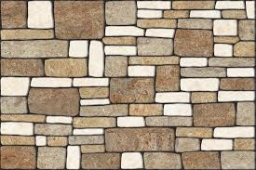# According 17163

According to the standard, seven tiles are able to pave the sidewalk in 12 shifts. After three shifts, one worker went on holiday. In how many shifts was the sidewalk paved?

n =  13.5

### Step-by-step explanation:

7·12 = 3·7 + 6·(n-3)

6n = 81

n = 81/6 = 13.5

n = 27/2 = 13.5

Our simple equation calculator calculates it.Did you find an error or inaccuracy? Feel free to write us. Thank you!

Tips for related online calculators
Do you have a linear equation or system of equations and looking for its solution? Or do you have a quadratic equation?
Do you want to convert time units like minutes to seconds?